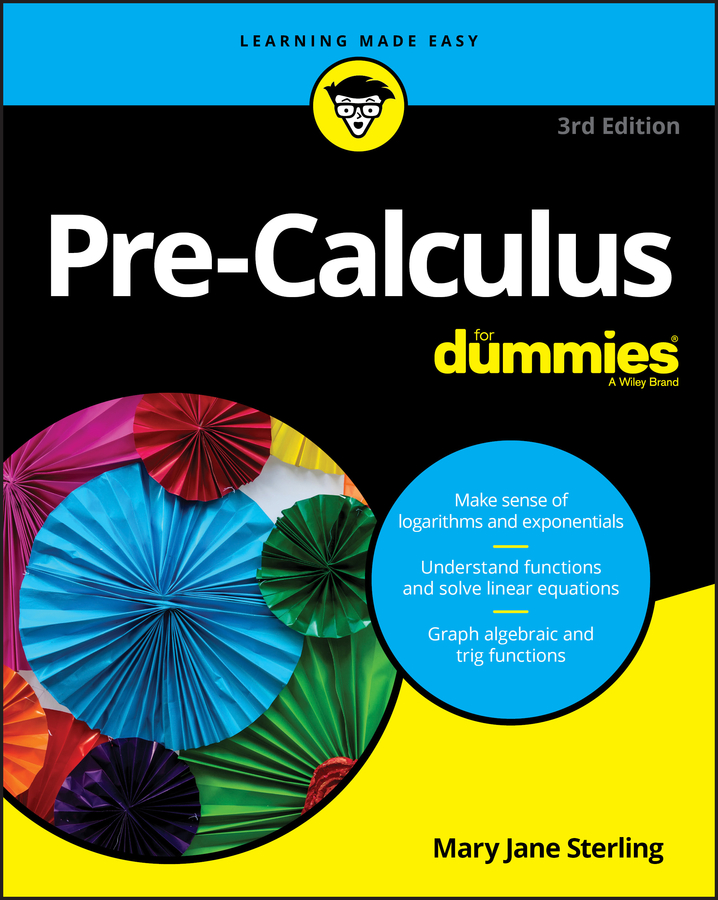##### Pre-Calculus For DummiesIf your pre-calculus teacher gives you two consecutive terms of an arithmetic sequence and asks you to find another, you can use a general formula to find the common difference between these terms. For example, an arithmetic sequence is –7, –4, –1, 2, 5. . . . If you want to find the 55th term of this arithmetic sequence, you can continue the pattern begun by the first few terms 50 more times. However, that process would be very time consuming and not very effective to find terms that come later in the sequence.

Instead, you can use a general formula to find any term of an arithmetic sequence. Finding the general formula for the nth term of an arithmetic sequence is easy as long as you know the first term and the common difference.

1. Find the common difference, d.

To find the common difference, simply subtract one term from the one after it: –4 – (–7) = 3. Thus d = 3.

2. Plug a1 and d into the general formula for any arithmetic sequence to write the specific formula for the given sequence.

1. an = a1 + (n – 1)d

Then plug in what you know: The first term of the sequence is –7, and the common difference is 3:

1. an = –7 + (n – 1)3 = –7 + 3n – 3 = 3n – 10

3. Plug in the number of the term you're trying to find for n.

To find the 55th term, plug 55 in for n into the general formula for an:

1. a55 = 3(55) – 10 = 165 – 10 = 155